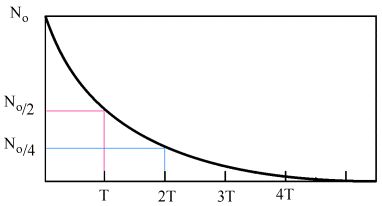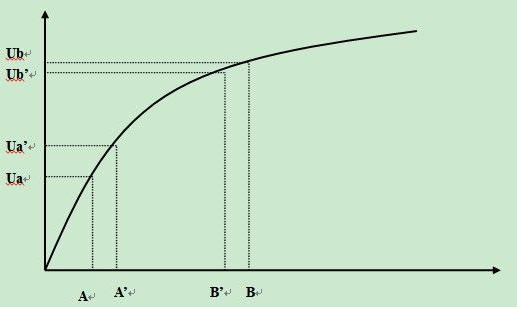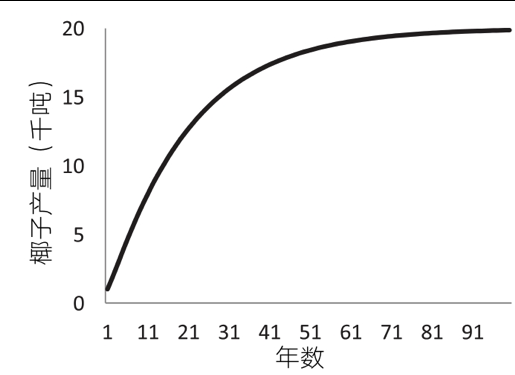## 凸函数$$V_t = V_0 (1+R)^t$$## 凹函数### 经济增长模型

C是常数, $\beta$ 是是介于0到1之间的实数，表示劳动力的相对重要性。在这里我们简单起见，使用1/2。我们可以把劳动力和资本结合起来看作一个机器M的产出,假设常数是100。这样在t时刻，$O_t= 100 \sqrt{M_t}$。我们有了一定的产出，之后我们可以将产出的部分进行储蓄，之后拿这部分再进行投资。同时我们使用的机器是有损耗的，用到一定时间就需要买新的。我们可以得到如下的方程

• 产出函数：$O_t= 100 \sqrt{M_t}$
• 投资规则：$I_t=s×O_t$
• 消费-投资方程：$O_t=C_t+I_t$
• 投资-折旧方程：$M_{t+1}=M_{t}+I_{t} - d × M_t$• 产出模型：$O_t = A_t K_t ^ {\beta} L_t ^ {1-\beta}$
• 长期均衡产出$O^\star = A_t^2 L \frac{s}{d}$